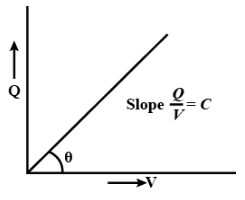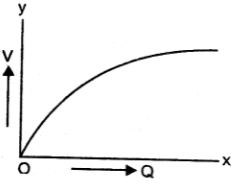Courses
Courses for Kids
Free study material
Offline Centres
MoreLast updated date: 03rd Dec 2023
Total views: 279.3k
Views today: 2.79k

# Sketch graph to show how $Q$ given to a capacitor $C$ charge varies with the potential difference.Verified
279.3k+ views
Hint: The ratio of the amount of electric charge deposited on a conductor to the difference in electric potential is known as capacitance. Self-capacitance and reciprocal capacitance are two closely related concepts of capacitance. Self-capacitance is a property of any material that can be electrically charged.

The electric potential difference between the target and the ground is determined in this situation. At a given potential difference, a substance with a high self capacitance carries more electric charge than one with a low capacitance. Knowledge of the action of the capacitor, one of the three basic linear electronic elements, requires an understanding of mutual capacitance (along with resistors and inductors).

The following is the relationship between the potential through the plates and the charge on the capacitor. As a result, we can see that V is proportional to $Q$. As a result, the graph must be a straight line from the origin with a positive slope.
$Q = C V$
It is of the form
$y=mx$
Here, $m=C$
$Q \propto V$The graph between $V$ and $Q$ is initially a straight line. However, after a certain point, charge leakage begins, and $V$ does not rise as $Q$ increases. The below figure depicts this difference.Note: Two conductors, one positively charged and the other negatively charged, are used to divide electric charge in a typical capacitor, but the circuit has a cumulative charge of zero. The amplitude of the electric charge on each conductor is the ratio in this case, and the potential differential is the difference determined between the two conductors.